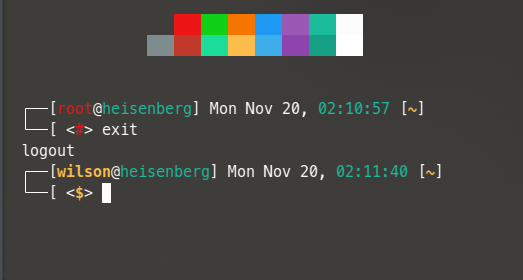Preferable in this format (so I can steal it others can recreate it):

• picture
• shell used
• prompt

Shamefully I still use the default prompt, but I will start anyway:

•• Bash
• `PS1='[\u@\h \W]\\$ '`
1 Like

I wish I could remember which bash prompt generator I used to do this.Bash shell

The one in /root has a different color for the user

``````2 line color prompt (root)
export PS1="┌──[\[\e[31m\]\u\[\e[m\]@\[\e[36m\]\h\[\e[m\]] \d, \[\e[36m\]\T\[\e[m\] [\e[1;33m\]\w\[\e[m\]] \n└──[ <\[\e[5;31m\]\\\$\[\e[m\]> "

2 line color prompt (user)
export PS1="┌──[\[\e[1;33m\]\u\[\e[m\]@\[\e[36m\]\h\[\e[m\]] \d, \[\e[36m\]\T\[\e[m\] [\e[1;33m\]\w\[\e[m\]] \n└──[ <\[\e[1;5;33m\]\\\$\[\e[m\]> "
``````
1 Like